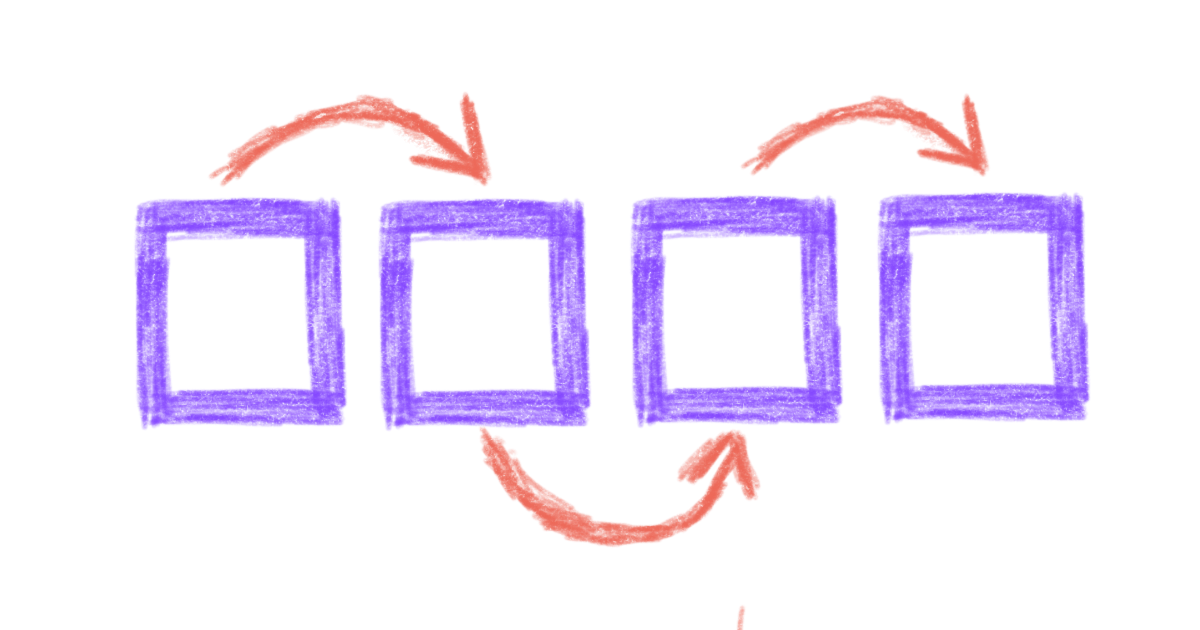# How to Write List Comprehensions with Python

One of the most common blocks you will write in Python scripts is a for loop. With for loops, you can repeat the same set of instructions in a block over and over. Python’s for loops are really foreach loops, where you repeat the instructions for every item in a collection. These collections are called iterators, which is something that a Python loop is able to iterate over, and the most common iterator is `list`.

## For Loops

Let’s look at an example of a for loop. Write a function that prints the square of each number from one to `n`:

``````def write_squares(n):
for number in range(n):
square = number ** 2
print(square)

write_squares(6)
``````

This is the output you will get from the above example:

``````0
1
4
9
16
25
``````

In `write_squares`, we calculate the variable `square` and print it for each value in `range(n)`, which is all of the numbers from `0` to `n` inclusive.Small for loops like this are very common in Python scripts. For example, we can read in lines from a file and strip any spaces from each line:

``````def lines_from(filename):
with open(filename, "r") as file_obj:

stripped_lines = []
for line in original_lines:
new_line = line.strip()
stripped_lines.append(new_line)
``````

Or, we might have a list of IDs and want to grab a piece of data for each ID:

``````def email_addresses(ids):
for identifier in ids:
data = api_request(identifier)
``````

With each of these for loops, we’re running the same piece of code on each item in the list. While the repeated piece of code isn’t too complicated, you still need to write (or read!) multiple lines to understand what the code block is doing. List comprehensions are an elegant way to create lists from existing lists.

## List Comprehension

First, let’s look at a quick example:

``````chars = [letter for letter in "Hello, world!"]
print(chars)
``````

When you run this Python code, the output will be:

``````['H', 'e', 'l', 'l', 'o', ',', ' ', 'w', 'o', 'r', 'l', 'd', '!']
``````

In this example, a new list is created, named `chars`, that contains each of the items in the string `"Hello, world!"`. Because items in a string are characters, `chars` is a list of every character in the string.

### List Comprehension Syntax

List comprehensions are written as:

``````new_list = [expression for item in old_list]
``````

This is what a list comprehension “unwrapped” into a traditional list would look like:

``````new_list = []
for item in old_list:
new_item = expression
new_list.append(new_item)
``````

Used correctly, list comprehensions can reduce for loops into a more readable, one line expression.### Examples

Let’s re-write our earlier examples using list comprehensions. Instead of writing an whole new function, our `write_squares` example can be reduced to one line:

``````squares = [number ** 2 for number in range(6)]
print(squares)
``````

We get the same results when we run this code:

``````0
1
4
9
16
25
``````

Now let’s look at the line stripping function. We need to keep the first few lines to read the file contents, but the for loop that appended each stripped line to a new variable has been updated to use a list comprehension instead:

``````def lines_from(filename):
with open(filename, "r") as file_obj:

return [line.strip() for line in original_lines]
``````

The email address fetcher can be reduced to one line, and it can be directly placed in another block of code:

``````def email_addresses(ids):
return [api_request(id)["email"] for id in ids]
``````

### Conditionals

List comprehensions can also use conditional statements to filter or modify the values that are added to a new list. For example, if we wanted a list of only even integers from 1 to 20, we could add a conditional to the end of a list comprehension:

``````>>> evens = [num for num in range(0, 20) if num % 2 == 0]
>>> print(evens)

[0, 2, 4, 6, 8, 10, 12, 14, 16, 18]
``````

List comprehensions can use any number of `and` and `or` operators in conditionals. For example, we can use the conditional `(num % 2 == 0) and (num % 3 == 0)` to keep only numbers that are divisible by both 2 and 3:

``````>>> my_nums = [num for num in range(0, 20) if (num % 2 == 0) and (num % 3 == 0)]
>>> print(my_nums)

[0, 6, 12, 18]
``````

## Key Points

List comprehension is an elegant way to create new lists from existing lists. List comprehensions can reduce multiple-line code blocks to just one line! However, avoid writing large list comprehensions, as that may reduce legibility for your code readers.

Once you’re comfortable with list comprehensions, I recommend learning dictionary comprehensions, which are very similar to list comprehensions except that they operate on dictionaries.